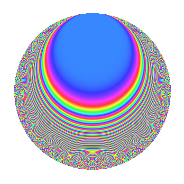Properties

 Label 1002.2.aLevel 1002 Weight 2 Character orbit a Rep. character $$\chi_{1002}(1,\cdot)$$ Character field $$\Q$$ Dimension 29 Newforms 11 Sturm bound 336 Trace bound 5

Related objects

Defining parameters

 Level: $$N$$ = $$1002 = 2 \cdot 3 \cdot 167$$ Weight: $$k$$ = $$2$$ Character orbit: $$[\chi]$$ = 1002.a (trivial) Character field: $$\Q$$ Newforms: $$11$$ Sturm bound: $$336$$ Trace bound: $$5$$ Distinguishing $$T_p$$: $$5$$

Dimensions

The following table gives the dimensions of various subspaces of $$M_{2}(\Gamma_0(1002))$$.

Total New Old
Modular forms 172 29 143
Cusp forms 165 29 136
Eisenstein series 7 0 7

The following table gives the dimensions of the cuspidal new subspaces with specified eigenvalues for the Atkin-Lehner operators and the Fricke involution.

$$2$$$$3$$$$167$$FrickeDim.
$$+$$$$+$$$$+$$$$+$$$$5$$
$$+$$$$+$$$$-$$$$-$$$$2$$
$$+$$$$-$$$$+$$$$-$$$$5$$
$$+$$$$-$$$$-$$$$+$$$$2$$
$$-$$$$+$$$$+$$$$-$$$$4$$
$$-$$$$+$$$$-$$$$+$$$$3$$
$$-$$$$-$$$$+$$$$+$$$$1$$
$$-$$$$-$$$$-$$$$-$$$$7$$
Plus space$$+$$$$11$$
Minus space$$-$$$$18$$

Trace form

 $$29q$$ $$\mathstrut +\mathstrut q^{2}$$ $$\mathstrut +\mathstrut q^{3}$$ $$\mathstrut +\mathstrut 29q^{4}$$ $$\mathstrut +\mathstrut 6q^{5}$$ $$\mathstrut +\mathstrut q^{6}$$ $$\mathstrut +\mathstrut 8q^{7}$$ $$\mathstrut +\mathstrut q^{8}$$ $$\mathstrut +\mathstrut 29q^{9}$$ $$\mathstrut +\mathstrut O(q^{10})$$ $$29q$$ $$\mathstrut +\mathstrut q^{2}$$ $$\mathstrut +\mathstrut q^{3}$$ $$\mathstrut +\mathstrut 29q^{4}$$ $$\mathstrut +\mathstrut 6q^{5}$$ $$\mathstrut +\mathstrut q^{6}$$ $$\mathstrut +\mathstrut 8q^{7}$$ $$\mathstrut +\mathstrut q^{8}$$ $$\mathstrut +\mathstrut 29q^{9}$$ $$\mathstrut +\mathstrut 2q^{10}$$ $$\mathstrut -\mathstrut 4q^{11}$$ $$\mathstrut +\mathstrut q^{12}$$ $$\mathstrut -\mathstrut 6q^{13}$$ $$\mathstrut -\mathstrut 8q^{14}$$ $$\mathstrut -\mathstrut 2q^{15}$$ $$\mathstrut +\mathstrut 29q^{16}$$ $$\mathstrut -\mathstrut 6q^{17}$$ $$\mathstrut +\mathstrut q^{18}$$ $$\mathstrut -\mathstrut 4q^{19}$$ $$\mathstrut +\mathstrut 6q^{20}$$ $$\mathstrut +\mathstrut 8q^{21}$$ $$\mathstrut +\mathstrut 4q^{22}$$ $$\mathstrut -\mathstrut 8q^{23}$$ $$\mathstrut +\mathstrut q^{24}$$ $$\mathstrut +\mathstrut 35q^{25}$$ $$\mathstrut +\mathstrut 6q^{26}$$ $$\mathstrut +\mathstrut q^{27}$$ $$\mathstrut +\mathstrut 8q^{28}$$ $$\mathstrut -\mathstrut 2q^{29}$$ $$\mathstrut +\mathstrut 2q^{30}$$ $$\mathstrut +\mathstrut 8q^{31}$$ $$\mathstrut +\mathstrut q^{32}$$ $$\mathstrut -\mathstrut 4q^{33}$$ $$\mathstrut +\mathstrut 2q^{34}$$ $$\mathstrut -\mathstrut 16q^{35}$$ $$\mathstrut +\mathstrut 29q^{36}$$ $$\mathstrut -\mathstrut 6q^{37}$$ $$\mathstrut -\mathstrut 12q^{38}$$ $$\mathstrut +\mathstrut 6q^{39}$$ $$\mathstrut +\mathstrut 2q^{40}$$ $$\mathstrut -\mathstrut 14q^{41}$$ $$\mathstrut +\mathstrut 8q^{42}$$ $$\mathstrut -\mathstrut 16q^{43}$$ $$\mathstrut -\mathstrut 4q^{44}$$ $$\mathstrut +\mathstrut 6q^{45}$$ $$\mathstrut -\mathstrut 8q^{46}$$ $$\mathstrut -\mathstrut 32q^{47}$$ $$\mathstrut +\mathstrut q^{48}$$ $$\mathstrut +\mathstrut 53q^{49}$$ $$\mathstrut -\mathstrut q^{50}$$ $$\mathstrut +\mathstrut 6q^{51}$$ $$\mathstrut -\mathstrut 6q^{52}$$ $$\mathstrut -\mathstrut 10q^{53}$$ $$\mathstrut +\mathstrut q^{54}$$ $$\mathstrut -\mathstrut 8q^{55}$$ $$\mathstrut -\mathstrut 8q^{56}$$ $$\mathstrut +\mathstrut 4q^{57}$$ $$\mathstrut +\mathstrut 30q^{58}$$ $$\mathstrut -\mathstrut 12q^{59}$$ $$\mathstrut -\mathstrut 2q^{60}$$ $$\mathstrut +\mathstrut 6q^{61}$$ $$\mathstrut +\mathstrut 8q^{63}$$ $$\mathstrut +\mathstrut 29q^{64}$$ $$\mathstrut -\mathstrut 12q^{65}$$ $$\mathstrut +\mathstrut 4q^{66}$$ $$\mathstrut -\mathstrut 16q^{67}$$ $$\mathstrut -\mathstrut 6q^{68}$$ $$\mathstrut +\mathstrut 8q^{69}$$ $$\mathstrut +\mathstrut 8q^{70}$$ $$\mathstrut -\mathstrut 24q^{71}$$ $$\mathstrut +\mathstrut q^{72}$$ $$\mathstrut +\mathstrut 26q^{73}$$ $$\mathstrut -\mathstrut 2q^{74}$$ $$\mathstrut +\mathstrut 15q^{75}$$ $$\mathstrut -\mathstrut 4q^{76}$$ $$\mathstrut -\mathstrut 32q^{77}$$ $$\mathstrut -\mathstrut 6q^{78}$$ $$\mathstrut -\mathstrut 16q^{79}$$ $$\mathstrut +\mathstrut 6q^{80}$$ $$\mathstrut +\mathstrut 29q^{81}$$ $$\mathstrut -\mathstrut 6q^{82}$$ $$\mathstrut -\mathstrut 20q^{83}$$ $$\mathstrut +\mathstrut 8q^{84}$$ $$\mathstrut -\mathstrut 12q^{85}$$ $$\mathstrut +\mathstrut 4q^{86}$$ $$\mathstrut -\mathstrut 18q^{87}$$ $$\mathstrut +\mathstrut 4q^{88}$$ $$\mathstrut -\mathstrut 22q^{89}$$ $$\mathstrut +\mathstrut 2q^{90}$$ $$\mathstrut +\mathstrut 24q^{91}$$ $$\mathstrut -\mathstrut 8q^{92}$$ $$\mathstrut +\mathstrut 32q^{93}$$ $$\mathstrut -\mathstrut 24q^{95}$$ $$\mathstrut +\mathstrut q^{96}$$ $$\mathstrut -\mathstrut 6q^{97}$$ $$\mathstrut +\mathstrut 9q^{98}$$ $$\mathstrut -\mathstrut 4q^{99}$$ $$\mathstrut +\mathstrut O(q^{100})$$

Decomposition of $$S_{2}^{\mathrm{new}}(\Gamma_0(1002))$$ into irreducible Hecke orbits

Label Dim. $$A$$ Field CM Traces A-L signs $q$-expansion
$$a_2$$ $$a_3$$ $$a_5$$ $$a_7$$ 2 3 167
1002.2.a.a $$1$$ $$8.001$$ $$\Q$$ None $$-1$$ $$-1$$ $$0$$ $$0$$ $$+$$ $$+$$ $$-$$ $$q-q^{2}-q^{3}+q^{4}+q^{6}-q^{8}+q^{9}+\cdots$$
1002.2.a.b $$1$$ $$8.001$$ $$\Q$$ None $$-1$$ $$-1$$ $$3$$ $$3$$ $$+$$ $$+$$ $$-$$ $$q-q^{2}-q^{3}+q^{4}+3q^{5}+q^{6}+3q^{7}+\cdots$$
1002.2.a.c $$1$$ $$8.001$$ $$\Q$$ None $$-1$$ $$1$$ $$-1$$ $$-1$$ $$+$$ $$-$$ $$-$$ $$q-q^{2}+q^{3}+q^{4}-q^{5}-q^{6}-q^{7}+\cdots$$
1002.2.a.d $$1$$ $$8.001$$ $$\Q$$ None $$-1$$ $$1$$ $$2$$ $$-4$$ $$+$$ $$-$$ $$-$$ $$q-q^{2}+q^{3}+q^{4}+2q^{5}-q^{6}-4q^{7}+\cdots$$
1002.2.a.e $$1$$ $$8.001$$ $$\Q$$ None $$1$$ $$1$$ $$-3$$ $$-3$$ $$-$$ $$-$$ $$+$$ $$q+q^{2}+q^{3}+q^{4}-3q^{5}+q^{6}-3q^{7}+\cdots$$
1002.2.a.f $$2$$ $$8.001$$ $$\Q(\sqrt{2})$$ None $$-2$$ $$-2$$ $$-4$$ $$0$$ $$+$$ $$+$$ $$+$$ $$q-q^{2}-q^{3}+q^{4}+(-2+\beta )q^{5}+q^{6}+\cdots$$
1002.2.a.g $$3$$ $$8.001$$ 3.3.1300.1 None $$-3$$ $$-3$$ $$3$$ $$1$$ $$+$$ $$+$$ $$+$$ $$q-q^{2}-q^{3}+q^{4}+(1-\beta _{1})q^{5}+q^{6}+\cdots$$
1002.2.a.h $$3$$ $$8.001$$ 3.3.148.1 None $$3$$ $$-3$$ $$-3$$ $$-5$$ $$-$$ $$+$$ $$-$$ $$q+q^{2}-q^{3}+q^{4}+(-1+\beta _{2})q^{5}-q^{6}+\cdots$$
1002.2.a.i $$4$$ $$8.001$$ 4.4.2777.1 None $$4$$ $$-4$$ $$5$$ $$1$$ $$-$$ $$+$$ $$+$$ $$q+q^{2}-q^{3}+q^{4}+(1-\beta _{1})q^{5}-q^{6}+\cdots$$
1002.2.a.j $$5$$ $$8.001$$ 5.5.11256624.1 None $$-5$$ $$5$$ $$-1$$ $$9$$ $$+$$ $$-$$ $$+$$ $$q-q^{2}+q^{3}+q^{4}+\beta _{2}q^{5}-q^{6}+(2+\cdots)q^{7}+\cdots$$
1002.2.a.k $$7$$ $$8.001$$ $$\mathbb{Q}[x]/(x^{7} - \cdots)$$ None $$7$$ $$7$$ $$5$$ $$7$$ $$-$$ $$-$$ $$-$$ $$q+q^{2}+q^{3}+q^{4}+(1-\beta _{1})q^{5}+q^{6}+\cdots$$

Decomposition of $$S_{2}^{\mathrm{old}}(\Gamma_0(1002))$$ into lower level spaces

$$S_{2}^{\mathrm{old}}(\Gamma_0(1002)) \cong$$ $$S_{2}^{\mathrm{new}}(\Gamma_0(167))$$$$^{\oplus 4}$$$$\oplus$$$$S_{2}^{\mathrm{new}}(\Gamma_0(334))$$$$^{\oplus 2}$$$$\oplus$$$$S_{2}^{\mathrm{new}}(\Gamma_0(501))$$$$^{\oplus 2}$$